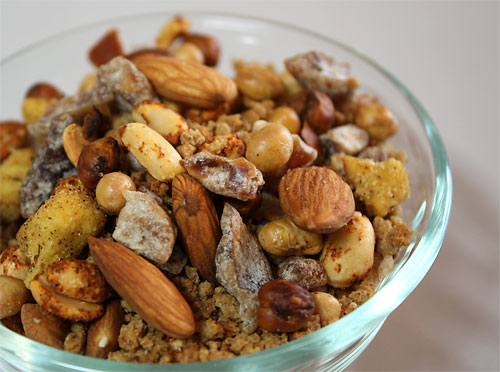Learn the 4 Things You Must Know Before Taking the GMAT!   Download it Now!Mixture problems (dry or chemical) are a common type of word problem tested on the GMAT. They all work essentially the same way and are relatively easy once you know what you’re supposed to do. In my video lesson “Problem Solving — Common GMAT Word Problems,” I teach a non-traditional strategy for solving mixture problems that is generally faster, easier, and more efficient than solving them the algebraic way. The benefit of that approach is that it saves students precious time on test day, and helps them to avoid wrong answers. However, I occasionally have students who ask for the algebraic approach anyway. Well, here you go. (I guess it’s not a bad idea to have an alternate approach in your back pocket in case you get stuck on test day!)

Consider this example:

Question: A dealer wishes to mix 20 pounds of nuts selling for 45 cents per pound with some more expensive nuts selling for 60 cents per pound to make a mixture that will sell for 50 cents per pound. How many pounds of the more expensive nuts should be used?

What to do? Well, if you’re trying to make your 10th grade algebra teacher happy and you really want to do this problem the algebraic way, then you’ll have to set up an algebraic expression and ultimately solve for x (where x represents the additional pounds of the more expensive nuts that should be used), right? The difficulty is, how do you set up that equation?

The secret to solving mixture problems the algebraic way is to make a table. In this example, the table would look like this:

 No. of lbs. *  Price / lb. =  Total Value Original 20 .45 .45 (20) Added x .60 .60 (x) Mixture 20 + x .50 .50 (20 + x)

The key here is to understand that the value of the original nuts plus the value of the additional nuts must equal the value of the mixture. Essentially, it’s just a giant weighted average problem. In almost all cases when you use a table to solve mixture problems the algebraic way on the GMAT, therefore, your equation simply comes from adding the final column:

.45(20) + .60(x) = .50(20+x)

At this point, the math is easy. Don’t forget to first multiply both sides of the equation by 100 to get rid of the decimals. The equation now becomes:

45(20) + 60(x) = 50(20+x)
900 + 60x = 1000 + 50x
10x = 100
x = 10

So, the answer is that the dealer should add 10lbs of 60-cent nuts to create the mixture he’s looking for.

Hopefully you can see how this approach would work for chemical (wet) mixtures as well.

If you prefer algebra and resonate with this methodology, then practice it until it becomes easy for you on all types of mixture problems. I hope it serves you well and enables you to go out an dominate the GMAT!